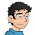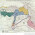## Wednesday, October 07, 2020

### Proportionality

In the list of consecutive positive base-ten integers: 1, 2, 3, ...

the 1111111111st occurrence of 1 is the first 1 in 1111111111,
the 2222222222nd occurrence of 2 is the second 2 in 2222222222,
the 3333333333rd occurrence of 3 is the third 3 in 3333333333,
the 4444444444th occurrence of 4 is the fourth 4 in 4444444444,
the 5555555555th occurrence of 5 is the fifth 5 in 5555555555,
the 6666666666th occurrence of 6 is the sixth 6 in 6666666666,
the 7777777777th occurrence of 7 is the seventh 7 in 7777777777,
the 8888888888th occurrence of 8 is the eighth 8 in 8888888888, and
the 9999999999th occurrence of 9 is the ninth 9 in 9999999999.

In other bases, the n-th occurrence of 1 as the first 1 in n is given in A023037 as a base-ten equivalent. Be careful about the offset (base two is the third term). Multiply the number by d for the n-th occurrence of d as the d-th d in n, up to d = base - 1. These n-th occurrence in n assertions are special solutions to the more general problem but they are not the only ones (all base-ten solutions are illustrated here).

1.Intriguing! And what about the 11th, 111th... occurrence of 1, the 22th, 222th... occurrence of 2? And in other bases, e.g., 11-th, 111-th... occurrence of 1 in numbers written in base 2, etc?

1.Yes, lots of room for discovery. I had actually done your 2nd, 22nd, 222nd, ... occurrences of 2: They happen in 12, 112, 612, 4112, 30612, 280182 (#2), 2615519, 24480182 (#2), 230744019, and 2222222222 (#2). I am too busy at the moment with other projects to explore any of this further.

2.Extending the original sequence to bases of 10, 11 etc., surely you get similar results about the AAAAA...th occurrence of A, where A means ten in base 11

3.For base b, there would be b-1 true assertions. In base two, the 3rd occurrence of 1 is the first 1 in 3. In base eleven, the final assertion would be that the 285311670610th occurrence of digit 10 is the tenth digit 10 in 285311670610. [Letting digit 10 = A: The AAAAAAAAAAAth occurrence of A is the Ath A in AAAAAAAAAAA.]